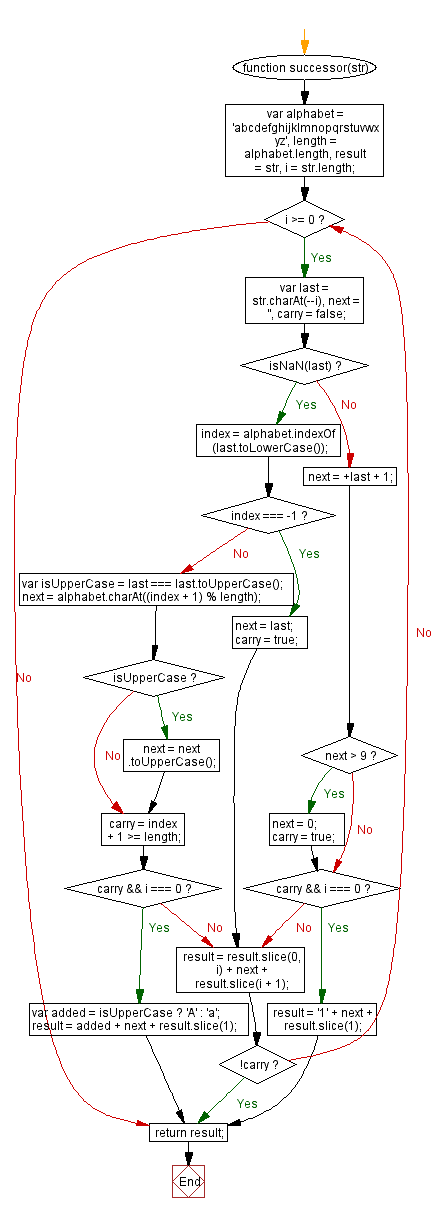# JavaScript: Get the successor of a string

## JavaScript String: Exercise-48 with Solution

Write a JavaScript function to get the successor of a string.

Note: The successor is calculated by incrementing characters starting from the rightmost alphanumeric (or the rightmost character if there are no alphanumerics) in the string. Incrementing a digit always results in another digit, and incrementing a letter results in another letter of the same case. If the increment generates a carry, the character to the left of it is incremented. This process repeats until there is no carry, adding an additional character if necessary.

Example:
string.successor("abcd") == "abce"
string.successor("THX1138") == "THX1139"
string.successor("< >") == "< >"
string.successor("1999zzz") == "2000aaa"
string.successor("ZZZ9999") == "AAAA0000"

Test Data:
console.log(successor('abcd'));
console.log(successor('3456'));
"abce"
"3457"

Sample Solution:-

HTML Code:

``````<!DOCTYPE html>
<html>
<meta charset="utf-8">
<title>JavaScript function to get the successor of a string</title>
<body>

</body>
</html>
```
```

JavaScript Code:

``````function successor(str) {
var alphabet = 'abcdefghijklmnopqrstuvwxyz',
length = alphabet.length,
result = str,
i = str.length;

while(i >= 0) {
var last = str.charAt(--i),
next = '',
carry = false;

if (isNaN(last)) {
index = alphabet.indexOf(last.toLowerCase());

if (index === -1) {
next = last;
carry = true;
}
else {
var isUpperCase = last === last.toUpperCase();
next = alphabet.charAt((index + 1) % length);
if (isUpperCase) {
next = next.toUpperCase();
}

carry = index + 1 >= length;
if (carry && i === 0) {
var added = isUpperCase ? 'A' : 'a';
result = added + next + result.slice(1);
break;
}
}
}
else {
next = +last + 1;
if(next > 9) {
next = 0;
carry = true;
}

if (carry && i === 0) {
result = '1' + next + result.slice(1);
break;
}
}

result = result.slice(0, i) + next + result.slice(i + 1);
if (!carry) {
break;
}
}
return result;
}

console.log(successor('abcd'));
console.log(successor('3456'));
```
```

Sample Output:

```abce
3457
```

Flowchart:Live Demo:

See the Pen JavaScript Get the successor of a string-string-ex-48 by w3resource (@w3resource) on CodePen.

Improve this sample solution and post your code through Disqus

What is the difficulty level of this exercise?

﻿

## JavaScript: Tips of the Day

JavaScript: Implicit type coercion

```function sum(a, b) {
return a + b;
}

sum(1, '2');
```

JavaScript is a dynamically typed language: we don't specify what types certain variables are. Values can automatically be converted into another type without you knowing, which is called implicit type coercion. Coercion is converting from one type into another.

In this example, JavaScript converts the number 1 into a string, in order for the function to make sense and return a value. During the addition of a numeric type (1) and a string type ('2'), the number is treated as a string. We can concatenate strings like "Hello" + "World", so what's happening here is "1" + "2" which returns "12".

Ref: https://bit.ly/323Y0P6